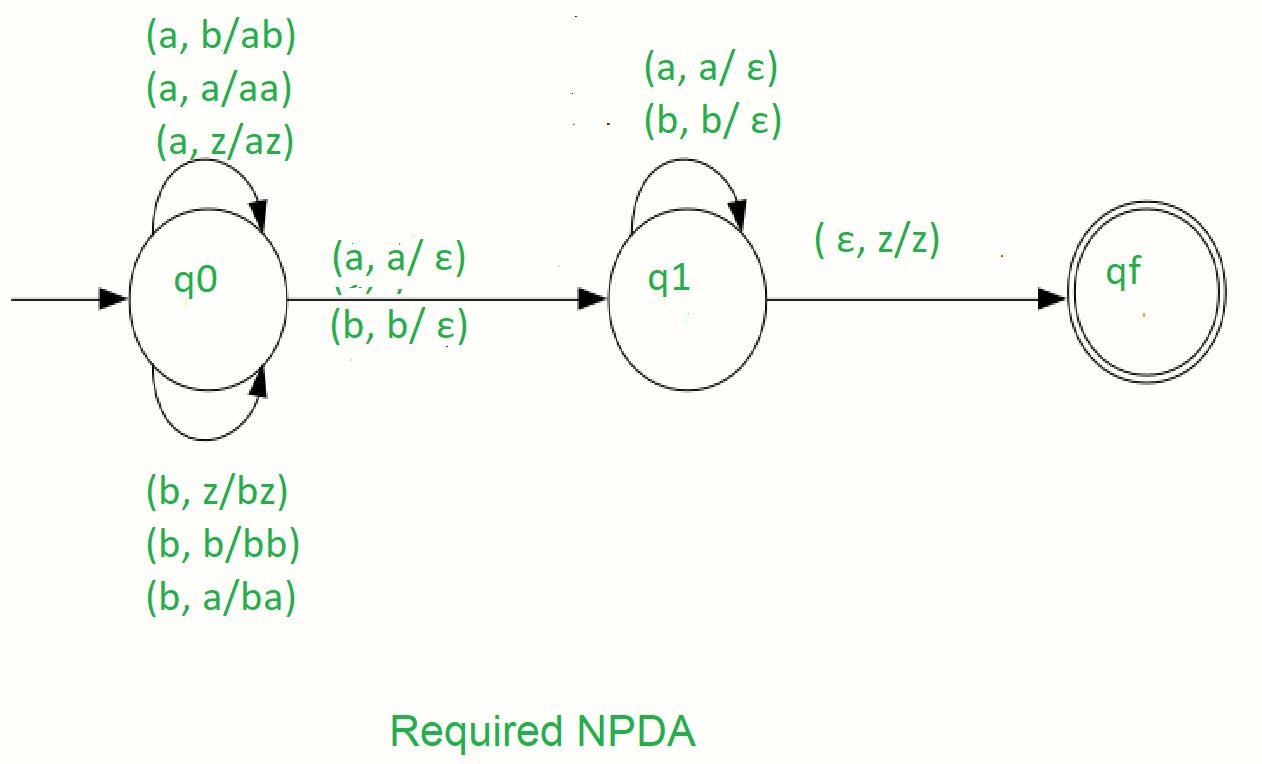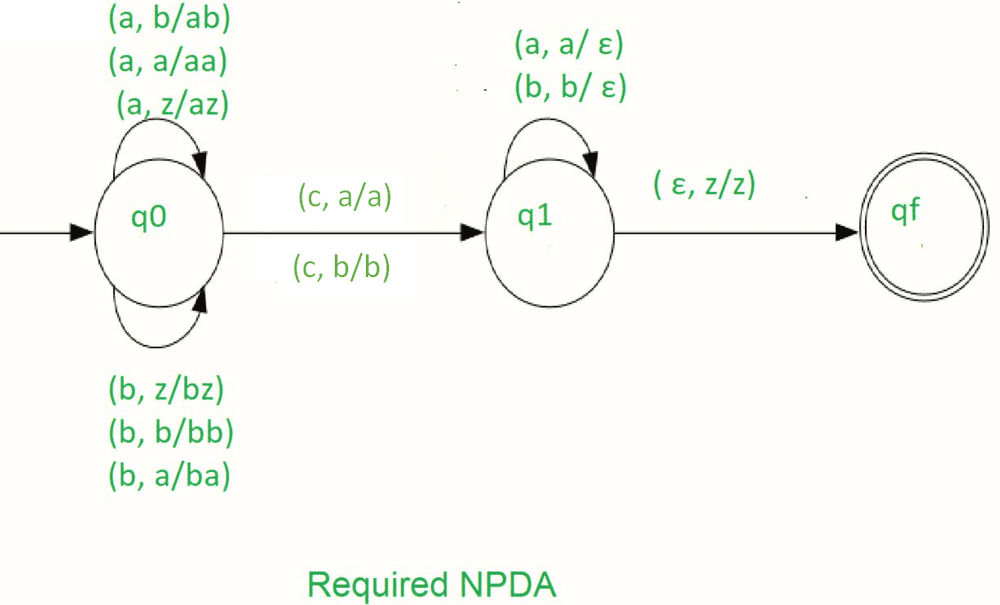# NPDA for accepting the language L = {wwR | w ∈ (a,b)*}

• Difficulty Level : Medium
• Last Updated : 18 Jul, 2022

Problem: Design a non deterministic PDA for accepting the language L = {wwR w ∈ (a, b)+}, i.e.,

L = {aa, bb, abba, aabbaa, abaaba, ......}

Prerequisite – Pushdown Automata, Pushdown Automata Acceptance by Final State

Explanation: In this type of input string, one input has more than one transition states, hence it is called non-deterministic PDA, and the input string contain any order of ‘a’ and ‘b’. Each input alphabet has more than one possibility to move next state. And finally when the stack is empty then the string is accepted by the NPDA. In this NPDA we used some symbols which are given below:

Γ = { a, b, z }

Where, Γ = set of all the stack alphabet
z = stack start symbol
a = input alphabet
b = input alphabet

The approach used in the construction of PDA –

As we want to design an NPDA, thus every times ‘a’ or ‘b’ comes then either push into the stack or move into the next state. It is dependent on a string. When we see the input alphabet which is equal to the top of the stack then that time pop operation applies on the stack and move to the next step.
So, in the end, if the stack becomes empty then we can say that the string is accepted by the PDA.

### STACK Transition Function(q0, a, z)(q0, az)(q0, a, a)(q0, aa)(q0, b, z)(q0, bz)(q0, b, b)(q0, bb)(q0, a, b)(q0, ab)(q0, b, a)(q0, ba)(q0, a, a)(q1, ∈)(q0, b, b)(q1, ∈)(q1, a, a)(q1, ∈)(q1, b, b)(q1, ∈)(q1, ∈, z)(qf, z)

Where, q0 = Initial state
qf = Final state
∈ = indicates pop operationSo, this is our required non-deterministic PDA for accepting the language L = {wwR w ∈ (a, b)*}

#### Example:

We will take one input string: “abbbba”.

• Scan string from left to right
• The first input is ‘a’ and follows the rule:
• on input ‘a’ and STACK alphabet Z, push the two ‘a’s into STACK as: (a, Z/aZ) and state will be q0
• on input ‘b’ and STACK alphabet ‘a’, push the ‘b’ into STACK as: (b, a/ba) and state will be q0
• on input ‘b’ and STACK alphabet ‘b’, push the ‘b’ into STACK as: (b, b/bb) and state will be q0
• on input ‘b’ and STACK alphabet ‘b’ (state is q1), pop one ‘b’ from STACK as: (b, b/∈) and state will be q1
• on input ‘b’ and STACK alphabet ‘b’ (state is q1), pop one ‘b’ from STACK as: (b, b/∈) and state will be q1
• on input ‘a’ and STACK alphabet ‘a’ and state q1, pop one ‘a’ from STACK as: (a, a/∈) and state will remain q1
• on input ∈ and STACK alphabet Z, go to the final state(qf) as : (∈, Z/Z)

So, at the end the stack becomes empty then we can say that the string is accepted by the PDA.

NOTE: This DPDA will not accept the empty language.

#### Problem:

Design a deterministic PDA for accepting the language L = { wcwR w ∈ (a, b)*}, i.e.,

{aca, bcb, abcba, abacaba, aacaa, bbcbb, .......}

In each string, the substring which is present on the left side of c is the reverse of the substring which is the present right side of c.

#### Explanation:

Here we need to maintain string in such a way that, the substring which is present on the left side of c is exactly the reverse substring which is the right side of c. For doing this we used a stack. In string ‘a’ and ‘b’ are present any order and ‘c’ come only one time. When ‘c’ comes then the pop operation is started into the stack. And when a stack is empty then language is accepted.

Γ = {a, b, z}

Where, Γ = set of all the stack alphabet
z = stack start symbol
a = input alphabet
b = input alphabet

#### The approach used in the construction of PDA:

As we want to design PDA In every time when ‘a’ or ‘b’ comes we push into the stack and stay on the same state q0. And when ‘c’ comes then we move to the next state q1 without pushing ‘c’ into the stack. And after when comes an input which is the same as the top of the stack then pop from the stack and stay on the same state. POP operation is performed until the input string is ended. Finally when the input is ∈, then move to the final state qf.
When if the stack will become empty then the language is accepted.

Where, q0 = Initial state
qf = Final state
z = stack start symbol
∈= indicates pop operation

#### Stack transition functions:(q0, a, z)(q0, az)(q0, a, a)(q0, aa)(q0, b, z)(q0, bz)(q0, b, b)(q0, bb)(q0, a, b)(q0, ab)(q0, b, a)(q0, ba)(q0, c, a)(q1, a)(q0, c, b)(q1, b)(q1, a, a)(q1, ∈)(q1, b, b)(q1, ∈)(q1, ∈, z)(qf, z)

Where, q0 = Initial state
qf = Final state
∈ = indicates pop operationSo, this is our required deterministic PDA for accepting the language,

L = { wcwR w ∈ (a, b)*}

My Personal Notes arrow_drop_up